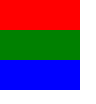# ObjectModel

Defines a set of items to be used as a model. More...

 Import Statement: import QtQml.Models

## Detailed Description

An ObjectModel contains the visual items to be used in a view. When an ObjectModel is used in a view, the view does not require a delegate since the ObjectModel already contains the visual delegate (items).

An item can determine its index within the model via the index attached property.

The example below places three colored rectangles in a ListView.

```import QtQuick 2.0
import QtQml.Models 2.1

Rectangle {
ObjectModel {
id: itemModel
Rectangle { height: 30; width: 80; color: "red" }
Rectangle { height: 30; width: 80; color: "green" }
Rectangle { height: 30; width: 80; color: "blue" }
}

ListView {
anchors.fill: parent
model: itemModel
}
}```## Property Documentation

The number of items in the model. This property is readonly.

## Attached Property Documentation

 ObjectModel.index : int

This attached property holds the index of this delegate's item within the model.

It is attached to each instance of the delegate.

## Method Documentation

 `[since 5.6]` append(object item)

Appends a new item to the end of the model.

`objectModel.append(objectComponent.createObject())`

This method was introduced in Qt 5.6.

 `[since 5.6]` clear()

Clears all items from the model.

This method was introduced in Qt 5.6.

 `[since 5.6]` object get(int index)

Returns the item at index in the model. This allows the item to be accessed or modified from JavaScript:

```Component.onCompleted: {
objectModel.append(objectComponent.createObject())
console.log(objectModel.get(0).objectName);
objectModel.get(0).objectName = "first";
}```

The index must be an element in the list.

This method was introduced in Qt 5.6.

 `[since 5.6]` insert(int index, object item)

Inserts a new item to the model at position index.

`objectModel.insert(2, objectComponent.createObject())`

The index must be to an existing item in the list, or one past the end of the list (equivalent to append).

This method was introduced in Qt 5.6.

 `[since 5.6]` move(int from, int to, int n = 1)

Moves n items from one position to another.

The from and to ranges must exist; for example, to move the first 3 items to the end of the model:

`objectModel.move(0, objectModel.count - 3, 3)`

This method was introduced in Qt 5.6.

 `[since 5.6]` remove(int index, int n = 1)